# Intervalo entre quartis

• Study Reminders

We'll email you at these times to remind you to study

You can set up to 7 reminders per week

#### You're all set

We'll email you at these times to remind you to study

Monday

Tuesday

Wednesday

Thursday

Friday

Saturday

Sunday

• Nota de Estudos
• Rever Tópicos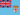Aisea K. 3 0 simple way of understanding basic concepts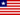Sylvester G G. 0 0 Good thanks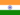N M. 0 0 nice wayof learningN M. 2 0 EASY TO UNDERSTANDN M. 0 0 thanks to alisonN M. 0 0 I'm enjoying it very much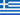Andreas M. 0 0 Thank you for the way of learning.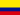Wilmar Alexander W. 0 0 The interquartile range is an appropriate measure of variability when used measure of central location was the median. It is defined as the difference between the third quartile (Q3 ) and the first quartile (Q1 ). RQ = Q3 - Q1.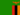George K. 0 0 why is not indicated how many temperatures in the lower half of 25%Batjargal P. 0 0 Calculation of the Inter-quartile Range We can use the sorted list to decide the median for the upper half and the median for the lower half of the original temperature data list. How many temperatures are there listed in September? Answer: 30 There are 15 temperature values in the upper half of the data. Which reading is the middle or median one for the upper half of the data (i.e. 7th or 8th or 9th highest)? Answer: 8th (15.1 degrees) What is the middle or median value in the upper half of the sorted list of temperatures? Answer: 15.1 What is the middle or median value in the lower half of the sorted list of temperatures? Answer: 11.2 What is the inter-quartile range for this group of temperatures? Answer: 3.9 degrees
• Text Version### Você recebeu uma nova notificação

Clique aqui para visualizar todos eles